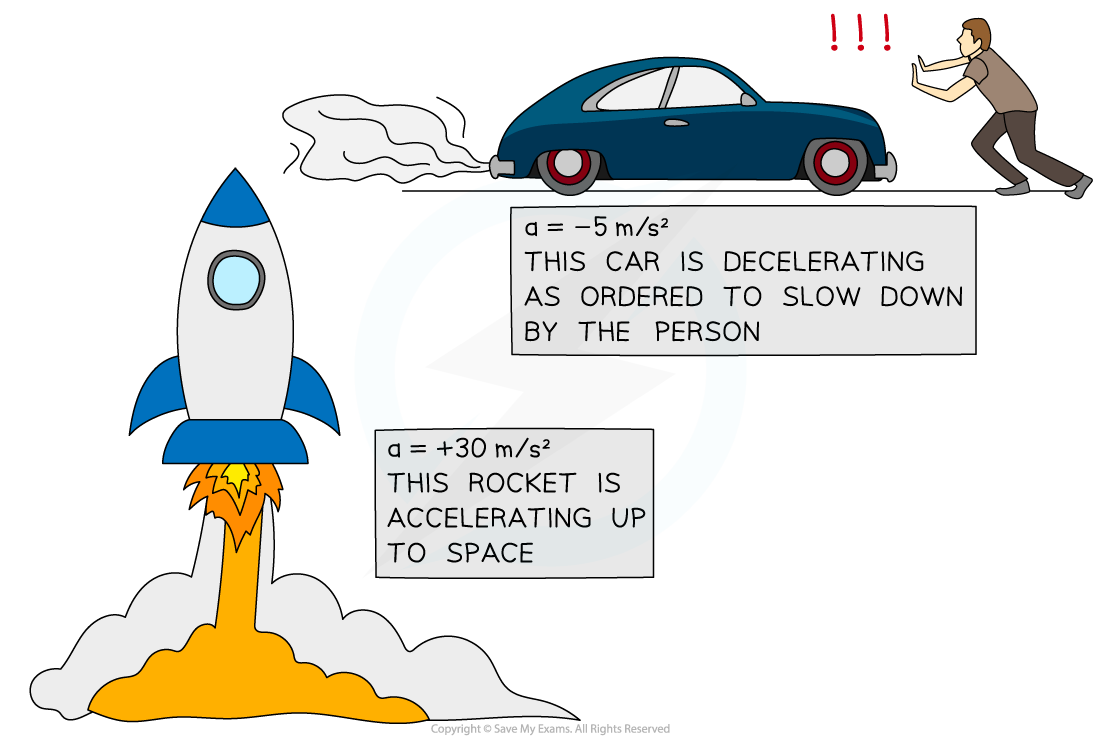# IB DP Physics: SL复习笔记2.1.3 Acceleration

### Acceleration

• Acceleration is defined as the rate of change of velocity
• In other words, it describes how much an object's velocity changes every second
• The equation below is used to calculate the average acceleration of an object:• Where:
• a = acceleration in metres per second squared (m s–2)
• Δv = change in velocity in metres per second (m s–1)
• t = time taken in seconds (s)
• The change in velocity is found by the difference between the initial and final velocity, as written below:

change in velocity = final velocity − initial velocity

#### Speeding Up and Slowing Down

• An object that speeds up is accelerating
• An object that slows down is decelerating
• The acceleration of an object can be positive or negative, depending on whether the object is speeding up or slowing down
• If an object is speeding up, its acceleration is positive
• If an object is slowing down, its acceleration is negative (sometimes called deceleration)A rocket speeding up (accelerating) and a car slowing down (decelerating)

#### Worked Example

A Japanese bullet train decelerates at a constant rate in a straight line.The velocity of the train decreases from 50 m s–1 to 42 m s–1 in 30 seconds.

(a) Calculate the change in velocity of the train.

(b) Calculate the deceleration of the train, and explain how your answer shows the train is slowing down.

Part (a)

Step 1: List the known quantities

• Initial velocity = 50 m s–1
• Final velocity = 42 m s–1

Step 2: Write the relevant equation

change in velocity = final velocity − initial velocity

Step 3: Substitute values for final and initial velocity

change in velocity = 42 − 50 = −8 m s–1

Part (b)

Step 1: List the known quantities

• Change in velocity, Δv = −8 m s–1
• Time taken, t = 30 s

Step 2: Write the relevant equationStep 3: Substitute the values for change in velocity and time

a = −8 ÷ 30 = −0.27 m s–1

Step 4: Interpret the value for deceleration

• The answer is negative, which indicates the train is slowing down

#### Exam Tip

Remember the units for acceleration are metres per second squared, m s–2In other words, acceleration measures how much the velocity (in m s–1) changes every second, m s–1 s–1.